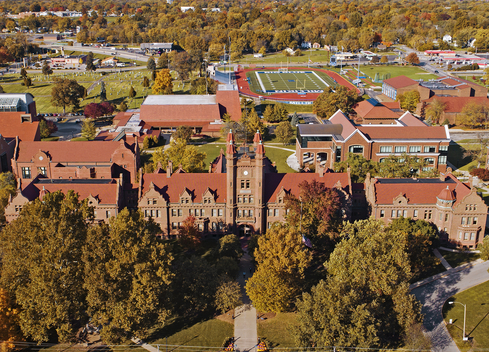# Computer Science Minor### Program Highlights

The Computer Science Minor offers students in all majors the opportunity to develop skills in writing algorithms to solve problems and to understand the fundamental concepts of computer science. This minor contains both theoretical and practical elements of the computer science field, with algorithm implementation and computability being prime examples. Further, the departmental goals of expressing and interpreting mathematical relationships from numeric, symbolic, and graphical points of view; reading and constructing mathematical proofs; analyzing various discrete and continuous probability models; and applying mathematics to other disciplines are directly addressed by this minor.

A student must complete a minimum of 21 credit hours.

### The following courses are required (15 credit hours)

• MA208 - Discrete Mathematics
• CS135 - Introduction to Computer Science
• CS235 - Introduction to Data Science
• CS308 - Theory of Computation
• CS337 - Algorithms and Data Structures

To complete the remaining 6 credit hour requirements, the student may choose two courses, one of which must bear a CS or IS prefix, from the following:

• CS300 - Advanced Discrete Mathematics
• CS342 - Artificial Intelligence
• IS221 - Programming Fundamentals
• IS321 - Systems Analysis and Design
• IS470 - Business Intelligence and Big Data
• MA420 - Mathematical Logic

Mathematics majors with a Data Science emphasis may not earn a Computer Science Minor. Note that some of these courses have prerequisites that need to be met. See course descriptions for details.

## Department News

•